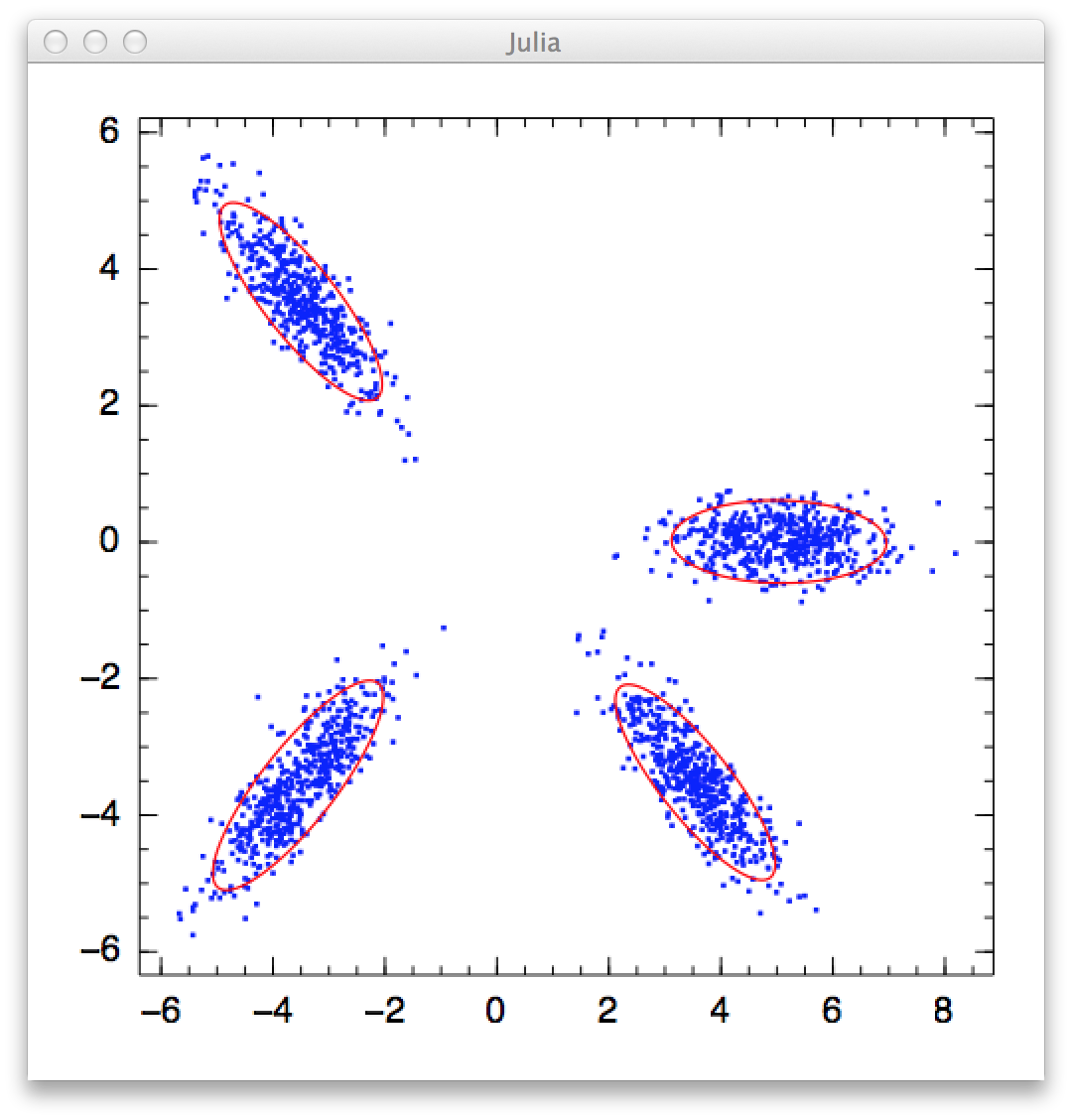# MixtureModels

12

6

5

1

## MixtureModels.jl

A Julia package for probabilistic mixture models

A mixture model is a probabilistic model that combines multiple components to capture data distribution. Generally, a mixture model is characterized by a collection of components and a discrete distribution over them. From a generative perspective, the procedure to generate a sample from a mixture model consists of two steps: (1) choose a particular component from the discrete distribution, and (2) use the chosen component to generate the sample.

## Finite Mixture Model

Finite mixture model is a classical formulation of mixture model, which requires the number of components, often denoted by K, to be fixed before training. The Expectation-Maximization (EM) algorithm is usually used to estimate a mixture model from data.

#### Basic Usage

The function `fit_fmm` fits a mixture model to a given data set:

``````fit_fmm(C, data, K, alg)
``````

Parameters:

• `C`: the component type, e.g. one can use `MultivariateNormal{PDMat}` to indicate Gaussian components with full covariance matrix.
• `data`: the input samples
• `K`: the number of components to be estimated
• `alg`: the algorithm option struct.

Here, `alg` can be constructed using the `fmm_em` function, as below

``````fmm_em(;
maxiter::Integer=100,    # maximum number of iterations
tol::Real=1.0e-6,        # tolerable change of objective value upon convergence
display::Symbol=:none,   # can take value :none, :proc, or :iter
alpha::Float64=1.0)      # Dirichlet prior coefficient for pi
``````

This function returns a struct of type `FiniteMixtureEMResults`, which contains the following fields:

• `mixture`: an instance of type `Mixture`, which has two fields: `components` (a list of components) and `pi` (component proportions);
• `Q`: soft assignment matrix, of size `(n, K)`. In particular, `Q[i, k]` indicates the probability that the i-th sample belongs to the k-th component;
• `L`: likelihood matrix, of size `(n, K)`. In particular, `L[i, k]` is the logpdf of the i-th sample w.r.t. the k-th component;
• `niters`: the number of elapsed iterations;
• `converged`: whether the procedure converged;
• `objective`: the objective function value of the last iteration.

#### Examples

``````# train a Gaussian mixture model with 5 components, showing progress information at each iteration
r = fit_fmm(MultivariateNormal{PDMat}, x, 5, fmm_em(maxiter=50, display=:iter))

# print each component
for comp in r.components
println(comp)
end

# If you are satisfied with default option values
r = fit_fmm(MultivariateNormal{PDMat}, x, 5, fmm_em())
``````

Note:

In Distributions.jl, the type `MultivariateNormal` takes a type argument that specifies the form of covariance matrix. Specifically, `MultivariateNormal{PDMat}` uses full covariance, `MultivariateNormal{PDiagMat}` uses diagonal covariance, while `MultivariateNormal{ScalMat}` uses a covariance of the form `s * I`.

The package provides a demo in `demo/gmm.jl` to demonstrate the use of this package in fitting Gaussian mixture model. Below is a screenshot of the result:#### Use User-supplied Q matrix

By default, this function initializes the soft assignment matrix `Q` randomly. The users can also provide their own version of initial Q-matrix, using the function `fit_fmm!`:

``````fit_fmm!(C, data, Q, alg)
``````

This function updates `Q` inplace.

This package provides a convenient function `qmatrix` to construct `Q`. For example, if you have a label vector `labels`, which may be obtained by running a clustering algorithm, you can use this vector to construct `Q`:

``````r = fit_fmm!(C, data, qmatrix(labels, K), alg)
``````

Here, `qmatrix` constructs a matrix `Q` of size `(n, K)` such that `Q[i, labels[i]] = 1.0` and each row sums to one.

## Future Plan

• Implement variants of Finite Mixture Models
• Implement Bayesian Nonparametric Mixture Models (e.g. DPMM)

07/19/2013